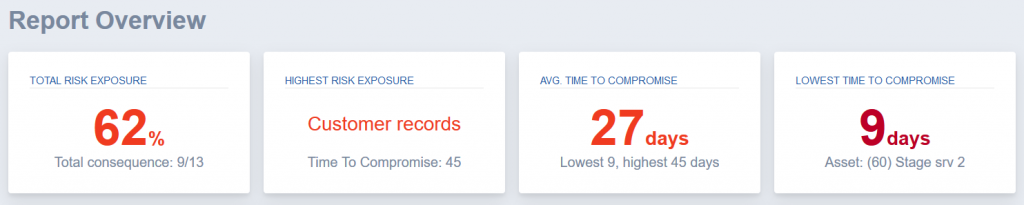# Report Overview Figures

At the very top of the report, we see some figures summarizing our risk exposure based on both the consequence values we have set and the Time To Compromise values securiCAD has calculated from the simulation results.Total Risk Exposure

The Total Risk Exposure value is a combination of consequence and TTC values. It is the main value of the risk exposure of the simulated model.

For the curious, the background to these figures are as follows;

• The consequence of Customer records / Write was set to 8 and the consequence of Stage srv 2 / Compromise was set to 5 in the model. This gives a sum of 13.
• The total TTC value (The Probability value in the High Value Assets table) was calculated by securiCAD to 58% and 68% respectively.
• This in turn means that the total risk exposure for Customer records / Write is 8 0,58 = 4,64 and for Stage srv 2 / Compromise, it is 5 0,68 = 3,4.
• Rounding these risk values upwards gives 4,64 + 3,4 as 5 + 4 which is shown as Total consequence: 9/13
• To present the total risk exposure value, the figures are not rounded which gives 4,64 + 3,4 = 8,04 and 8,04 / 13 = 0,62

Highest Risk Exposure

The Highest Risk Exposure box is showing the asset which according to the simulations and consequence values has got the highest risk exposure. In the case with the example model, it is the Customer records asset.

Avg Time To Compromise

Average, max and min values of the Time To Compromise figures.

Lowest Time To Compromise

For the example model, the High Value Asset with the lowest Time To Compromise value (of 9 days) is the Stage srv 2 object with object id number 60 in the model.

Summary

The figures, risk exposure values and Time To Compromise values for the selected High Value Assets are usually a good and digestible input to security assessment reports. What we typically present at an early stage when doing such reports are these risk exposure values and also what objects in the modeled architecture that has the highest risk exposure.

However, securiCAD does not only calculate these values based on attack simulations, but also allows you to follow the attack paths to see how an attacker is expected to go about when trying to reach the High Value Assets.

Reading the attack paths is described in the next module; Attack Paths.

What’s Next